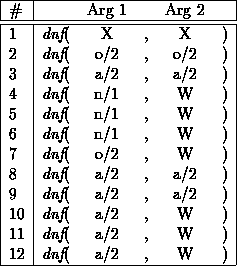Next: Benchmarks Up: Indexing PROLOG Procedures into Previous: User Commands

# Sample SessionIn order to show all index features of the compiler, we now want to introduce a larger example and the solutions after each compilation step.

The example is the dnf-procedurewhich produces the disjunctive normal form of a logic formula with the operators 'and', 'or' and 'not' (here written as a, o, and n).

We begin our example with the RELFUN program of dnfand its head information:

```
dnf(X, X) :- literal(X).
dnf(o[X, Y], o[X, Y]) :- literal(X), literal(Y).
dnf(a[X, Y], a[X, Y]) :- literal(X), literal(Y).
dnf(n[n[X]], W) :- dnf(X, W).
dnf(n[o[X, Y]], W) :- dnf(a[n[X], n[Y]], W).
dnf(n[a[X, Y]], W) :- dnf(o[n[X], n[Y]], W).
dnf(o[X, Y], W) :- dnf(X, X1), dnf(Y, Y1), norm(o[X1, Y1], W).
dnf(a[X, Y], a[a[X1, X2], Y]) :- literal(Y), dnf(X, a[X1, X2]).
dnf(a[X, Y], a[a[Y1, Y2], X]) :- literal(X), dnf(Y, a[Y1, Y2]).
dnf(a[X, Y], W) :- dnf(X, a[X1, X2]),
dnf(Y, a[Y1, Y2]),
norm(a[a[X1, X2], a[Y1, Y2]], W).
dnf(a[X, Y], W) :- dnf(X, o[X1, X2]),
dnf(Y, Y1),
dnf(o[a[X1, Y1], a[X2, Y1]], W).
dnf(a[X, Y], W) :- dnf(X, X1),
dnf(Y, o[Y1, Y2]),
dnf(o[a[X1, Y1], a[X1, Y2]], W).```Classified clauses (indexing part):

```
(proc
dnf/2
12
(indexing
(sblock
(rblock
(clauses 1 2 3 4 5 6 7
8 9 10 11 12)
(arg
1
(var x)
(struct o 2)
(struct a 2)
(struct n 1)
(struct n 1)
(struct n 1)
(struct o 2)
(struct a 2)
(struct a 2)
(struct a 2)
(struct a 2)
(struct a 2) )
(arg
2
(var x)
(struct o 2)
(struct a 2)
(var w)
(var w)
(var w)
(var w)
(struct a 2)
(struct a 2)
(var w)
(var w)
(var w) ) )
(seqind
(arg
1
(info 3)
(const)
(struct
((o 2)
(clauses 1 2 7)
(sblock
(rblock (clauses 1 2 7)
(arg
2
(var x)
(struct o 2)
(var w)))
(seqind
(arg
2
(info 1)
(const)
(struct ((o 2)
(clauses 1 2 7)))
(list)
(nil)
(other (clauses 1 7))))))
((a 2)
(clauses 1 3 8 9 10 11 12)
(sblock
(rblock
(clauses 1 3 8 9 10 11 12)
(arg
2
(var x)
(struct a 2)
(struct a 2)
(struct a 2)
(var w)
(var w)
(var w) ) )
(seqind
(arg
2
(info 1)
(const)
(struct ((a 2) (clauses 1 3 8 9 10 11 12)))
(list)
(nil)
(other (clauses 1 10 11 12))))))
((n 1)
(clauses 1 4 5 6)
(pblock
(rblock (clauses 1 4 5 6)
(arg
2
(var x)
(var w)
(var w)
(var w)))
(1block (clauses 1) (arg 2 (var x)))
(1block (clauses 4) (arg 2 (var w)))
(1block (clauses 5) (arg 2 (var w)))
(1block (clauses 6) (arg 2 (var w))))))
(list)
(nil)
(other (clauses 1)) )
(arg
2
(info 2)
(const)
(struct
((o 2) (clauses 1 2 4 5 6 7 10 11 12))
((a 2) (clauses 1 3 4 5 6 7 8 9 10 11 12)) )
(list)
(nil)
(other (clauses 1 4 5 6 7 10 11 12)) ) ) ) )
(fun*den  ; clauses part omitted
.....
))```

The indexing switches had the following values:

```
indexing on
:min-clauses 2
:max-vars 10
:max-depth 1
:max-args 2
:debug off```

In the following we abbreviate the constraints of the type-box in the index tree: c is the constant constraint, str is the structure constraint, l is the list constraint, n is the nil constraint, and the else constraint is the link on the right side of the box (without name).

The index tree corresponding to the index header of the classified dnf/2 clauses is of the following form:The resulting index codeis:

```
((set_index_number 1)
(switch_on_term 1 "label58" 1 1 "label50")
"label58"
(switch_on_structure
3
(((o 2) "label35") ((a 2) "label42") ((n 1) "label49"))
1 )
"label35"
(set_index_number 2)
(switch_on_term "label36" "label59" "label36" "label36" "label38")
"label59"
(switch_on_structure 1 (((o 2) "label38")) "label36")
"label36"
(try 1 2)
(trust 7 2)
"label38"
(try 1 2)
(retry 2 2)
(trust 7 2)
"label42"
(set_index_number 2)
(switch_on_term "label43" "label60" "label43" "label43" "label45")
"label60"
(switch_on_structure 1 (((a 2) "label45")) "label43")
"label43"
(try 1 2)
(retry 10 2)
(retry 11 2)
(trust 12 2)
"label45"
(try 1 2)
(retry 3 2)
(retry 8 2)
(retry 9 2)
(retry 10 2)
(retry 11 2)
(trust 12 2)
"label49"
(try 1 2)
(retry 4 2)
(retry 5 2)
(trust 6 2)
"label50"
(set_index_number 2)
(switch_on_term "label51" "label61" "label51" "label51" "label57")
"label61"
(switch_on_structure 2 (((o 2) "label53")
((a 2) "label54")) "label51")
"label57"
(try 1 2)
(retry 2 2)
(retry 3 2)
(retry 4 2)
(retry 5 2)
(retry 6 2)
(retry 7 2)
(retry 8 2)
(retry 9 2)
(retry 10 2)
(retry 11 2)
(trust 12 2)
"label53"
(try 1 2)
(retry 2 2)
(retry 4 2)
(retry 5 2)
(retry 6 2)
(retry 7 2)
(retry 10 2)
(retry 11 2)
(trust 12 2)
"label54"
(try 1 2)
(retry 3 2)
(retry 4 2)
(retry 5 2)
(retry 6 2)
(retry 7 2)
(retry 8 2)
(retry 9 2)
(retry 10 2)
(retry 11 2)
(trust 12 2)
"label51"
(try 1 2)
(retry 4 2)
(retry 5 2)
(retry 6 2)
(retry 7 2)
(retry 10 2)
(retry 11 2)
(trust 12 2))
1               ; WAM code for clauses omitted
....
2
....```

Michael Sintek - sintek@dfki.uni-kl.de